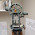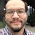## Friday, June 6, 2014

### HP 15C: Area of a Polygon by Vertices (revised)

HP 15C: Area of a Polygon by Vertices (revised)

In December 2011, I posted a program to calculate the area of a polygon given the vertices for the HP 15C. You can find the original program here:

http://edspi31415.blogspot.com/2011/12/hp-15c-programming-tutorial-part-15.html

The problem with this, that the area is not accurate when the initial point is not (0,0). This was brought up by Jason Foose. Jason, a million thanks.

Here is another attempt at it, this time it should work with any initial point. I tested the program with several different polygons and so far, so good.

Please test and let me know what you think. The instructions remain the same:
1. Enter the initial y coordinate, press ENTER, then enter the initial x coordinate, and press [ f ] [ √ ] (LBL A).
2. For each additional point, enter the y coordinate, press ENTER, then enter the x coordinate, and finally [ f ] [ e^x ] (LBL B).
3. To calculate the area, press [ f ] [10^x ] (LBL C).

Memory registers used:
R0 = area
R1 = x_i
R2 = y_i
R3 = x_i+1
R4 = y_i+1
R5 = Σ (x_i * y_i+1) // ( i=1...n and cycle back to 1)
R6 = Σ (x_i+1 * y_i)
R7 = x_1
R8 = y_1

Program - HP 15C:
LBL A
0
STO 5
STO 6
R down \\ R↓
STO 1
STO 7
R down
STO 2
STO 8
RTN

LBL B // step 12
STO 3
RCL× 2
STO+ 6
R down
STO 4
RCL× 1
STO+ 5
RCL 4
STO 2
RCL 3
STO 1
RTN

LBL C // step 25
RCL 7
RCL× 2
STO+ 6
RCL 8
RCL× 1
STO+ 5
RCL 5
RCL- 6
ABS
2
÷
STO 0
RTN

Total: 38 steps

Eddie

This blog is property of Edward Shore. 2014

#### 2 comments:

1.My sample data @ 5000,5000 irregular 4 sided polygon worked great! I took the liberty of creating a simple acreage addendum after line 37 (sto 0). Lines 038-042 represent the number 43560(sqft in an acre) and line 043 is divide. Acreage will be visible in the x-reg and sqft is easily accessible by rcl 0. Very handy program! Great work and thanks Eddie.

1.Thank you Jason! And thank you for pointing out the flaw in my original program.

Eddie

### HP Prime and HP 41C/DM 41L: Sum of Two Squares

HP Prime and HP 41C/DM 41L:  Sum of Two Squares  Introduction Given a positive integer n, can we find two non-negative integers x and y...